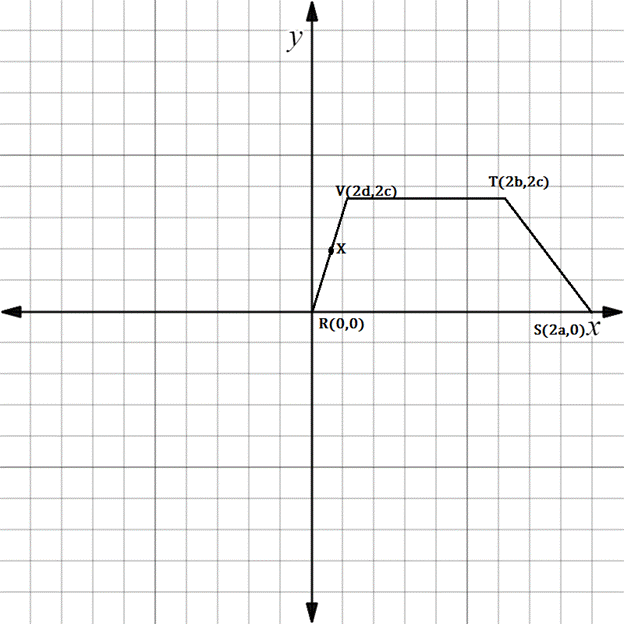Chapter 10.3, Problem 32EElementary Geometry For College St...

7th Edition
Alexander + 2 others
ISBN: 9781337614085

Solutions

Chapter
SectionElementary Geometry For College St...

7th Edition
Alexander + 2 others
ISBN: 9781337614085
Textbook Problem

Which formula would you use to establish each of the following claims?a) The coordinates of X are d ,   c .b) m V T - = 0 c) V T - ║ R S - d) The length of R V - is 2 d 2 + c 2Trapezoid R S T V ; X is the midpoint of R V - .

To determine

(a)

To find:

The formula used to find the coordinates of X.

Explanation

Since X is found midway between R and V.

Hence, midpoint formula is used

To determine

(b)

To find:

The formula used to establish mVT-=0.

To determine

(c)

To find:

The formula used to establish VT-RS-.

To determine

(d)

To find:

The formula used to establish the length of RV- is 2d2+c2.

Still sussing out bartleby?

Check out a sample textbook solution.

See a sample solution

The Solution to Your Study Problems

Bartleby provides explanations to thousands of textbook problems written by our experts, many with advanced degrees!

Get Started

Convert the expressions in Exercises 31-36 to positive exponent form. 10.3x265x1

Finite Mathematics and Applied Calculus (MindTap Course List)

Differentiate the function. f(x)=ln1x

Single Variable Calculus: Early Transcendentals, Volume I

Fill in each blank: 120ft=yd

Elementary Technical Mathematics

In problems 33-36, write an inequality that discribes each interval or graph. 36.

Mathematical Applications for the Management, Life, and Social Sciences

In Exercises 75-98, perform the indicated operations and/or simplify each expression. 78. 3(2a b) 4(b 2a)

Applied Calculus for the Managerial, Life, and Social Sciences: A Brief Approach